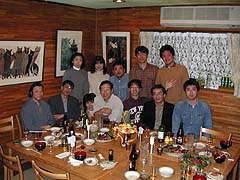# \$BBh(J33\$B2s(B \$B7nNc%m!<%t%#%8%g%s8&5f2q(J

(B (J\$B@nEh1Q;L!&>.ED9@0l(J,(B (J2000/12/(B9(J

(B (J
```\$B!!(B
\$BF|;~!'(J2000\$BG/(B (J12\$B7n(B (J9\$BF|(B (J(\$BEZ(J)\$B!!(J12\$B!'(J30-(B
\$B>l=j!'El5~=w;RBg3X>.ED8&5f<<(J(8\$B9f4[(J4\$B3,(J)(B
\$B!!(B
\$B!!(B
\$B!!(J```
(B (J

### \$B;22C!J8^==2;=g!K(J

(B (J
```\$B0BF#(B \$B?-O/!w:Q@82q?73cBhFsIB1!4c2J(B
\$BHx7A(B \$B??.ED(B \$B9@0l!wEl5~=w;RBg3X(B
\$B>.EDEg(B \$B909,!w%J%\$%D(B
\$B>.NS(B \$B>O!w9qN)?HBN>c32.NS(B \$B4`!w4dOF(B \$BM'5*!w0INS%"%\$%;%s%?!<(B
\$BD%BX(B \$BNC;R!w?73cBg3X4c2J(B
\$B>>ED(B \$B??9,!w:n?73X1!Bg3X(B
\$B>>K\(B \$BMF;R!w=Y2OBfF|K\Bg3XIB1!(B
(B (J

### \$B%W%m%0%i%`(J

(B (J
```1(B3(J:(B15(J-13:(B45
\$B>.NS(B \$B4`!w4d\$B!VJ?@.(J12\$BG/EY3\$30=PD%Js9p!W(B(PDF 45K)
\$B!!(B
(J13:(B50(J-1(B4(J:30(B
\$B>.NS(B \$B>O!w9qN)?HBN>c32\$B!V%k!<%Z\$NG\N(7W;;K!(J(6)\$B%l%s%:\$H4c\$N0LCVJQ2=\$KH<\$&G\N(\$NJQ2=!W(B
\$BG\N(\$NI=(B
\$B!!(B
(J1(B4(J:30-1(B5(J:00(B
\$B>>ED(B \$B??9,!w:n?73X1!Bg3X(B
\$B!VF|K\8lJ8\$NI=5-7ABV\$HFI\$_\$N4c5e1?F0!W(B
\$B!!(B
(J1(B5(J:00-1(B6(J:(B1(J0(B
\$BEDCf(B \$B7CDE;R!w0INS%"%\$%;%s%?!<(B
\$B!!(B
16:10-16:40 coffee break

(J1(B6(J:(B40(J-1(B7(J:(B25
\$B0BF#(B \$B?-O/!w:Q@82q?73cBhFsIB1!4c2J(B
\$B!VE|G"IBLVKl>I4IM}\$K\$*\$1\$kIB?GO"7H\$N\$"\$jJ}!W(B
\$BA49q\$NE|G"IB45.ED(B \$B9@0l!wEl5~=w;RBg3X(B
\$B!!(B
--- \$B2<5-\$NJ88%\$O\$5\$o\$j\$@\$1>R2p\$5\$l!"B3\$-\$O(B21\$B@\$5*:G=i\$N(B
\$B\$*G/6L\$H\$\$\$&\$3\$H\$K\$J\$j\$^\$7\$?!'(B
\$BHx7A(B \$B??(B
\$BK:G/2q(J\$B!!(B\$B!!(B
\$B>l=j!'(JPEIPAR(B (JBAN(B
\$B;~4V!'(J19:00\$B!A(B
\$B2qHq!'!o(J3,000\$B!!%W%i%9\$*!J(J50\$B2;=g!K(B
\$B!!(B
\$B0BF#(B \$B?-O/!w:Q@82q?73cBhFsIB1!4c2J(B
\$BHx7A(B \$B??.ED(B \$B9@0l(J@\$BEl5~=w;RBg3X(B
\$B>.EDEg(B \$B909,!w%J%\$%D(B
\$B>.NS(B \$B>O!w9qN)?HBN>c32.NS(B \$B4`!w4dOF(B \$BM'5*(J@\$B0INS%"%\$%;%s%?!<(B
\$B>>ED(B \$B??9,(J@\$B:n?73X1!Bg3X(B
\$B>>K\(B \$BMF;R!w=Y2OBfF|K\Bg3XIB1!(B